## "Tomography Problems at Monochromatic Sounding"

Valery P. Yushchenko*

Ministry of Education and Science of the Russian Federation, Novosibirsk State Technical University, Novosibirsk, Russia

*Corresponding author: Valery P. Yushchenko, Ministry of Education and Science of the Russian Federation, Novosibirsk State Technical University, 630073, Novosibirsk, K.Marx Avenue, 20, RussiaTel:+73832243421; Email: jwp@aport2000.ru

Received Date: 13 August, 2018; Accepted Date: 24 August, 2018; Published Date: 03 September, 2018

1.       Summary

In this article it is discussed methods of creation of the image of objects on the Doppler signal reflected from it by means of the synthesized antenna aperture. The known property of the synthesized aperture to be focused on the set range is the cornerstone of a method. Difference of the offered methods from the known methods that the focused spot scans interiors of object and is developed the image of object in section. Such chance is given by repeated correlation of the object signal received as a result of a Doppler location with basic trajectory signals for the dot objects received as a result of calculation. Cartograms of the model objects reconstructed on a Doppler signal are given. Problems of practical realization are discussed.

1.                   Introduction

Tomography is the most informative measuring process. The tomography assumes reconstruction of the image of object in section with display of internal structure of object. For a tomography it is necessary to choose the probing signals which get in inside the studied object. It can be the X-rays, radioactive radiations, microwave ovens radiation provoking a nuclear magnetic resonance in a gradient magnetic field, a radio emission and, at last, ultrasonic radiation.

All types of a tomography are useful. They supplement, but do not replace each other. Likely the most qualitative are nuclear magnetic resonances tomographs, however they are not deprived of shortcomings. The patient when diagnosing has to lie not movably within 30 minutes. For the patient with feeling sick it is not comfortable procedure.

Let's stop on ultrasonic radiation. It gets in inside object and at the small capacities is not capable to do harm to the patient. It is possible to make a critical remark that in an ultrasonic tomography it is difficult to think up something new. Besides ultrasonography tomographs are rather perfect. However, there are claims to results of ultrasonography. The received image is vague and its clearness is not sufficient. Figure 1.

Dispersive distortions are the reason for that. Fabrics of a live organism are the dispersive environment for an ultrasonic wave. The essence of these distortions consists that the short probing impulse has a wide range. In the dispersive environment components of a range extend with an unequal speed. It leads to the fact that the short impulse which passed through the dispersive environment is washed away (becomes wider and gets out of a shape). It does not allow to construct the accurate image.

2.                   Problem Definition

It is offered to refuse sounding by short impulses and to pass to the monochromatic probing signals. The range of such signal contains one frequency therefore there will be no dispersive distortions.

However, it is not simple to apply monochromatic signals in a location and in a tomography. Monochromatic signals have no radial permission. And it is necessary for reconstruction of the image. For a solution of the problem of radial permission we will use property of the synthesized aperture to be focused on the set range. The synthesized aperture is similar to a lens and has focal length.

To use synthesis of an aperture it is necessary to organize the movement of a locator concerning object at small distance on a rectilinear or circular trajectory with the known speed and to register a trajectory Doppler signal.

For a Tomography, it is supposed to use a one-position ultrasonic Doppler locator at which the radiating and accepting antennas are combined, that is approximately are in one point of space, and have a weak focus in the equatorial plane and a sharp orientation 6º in the meridional plane .

3.                   Description of Laboratory Installation

The function chart of a Doppler locator is submitted in Figure 2.

In Figure 2, the following designations are accepted: the 1-radiating piezo element 2-accepting a piezo element, 90º -a phase-shifting chain on 90º, CM-The Mixer of Signals (remultiplier), the Cut. Mustache.RA- The Resonant Amplifier, DA-The Amplifier Of Doppler Frequencies, ADC-The Analog-Digital Converter.

Installation allows to realize both rectilinear, and circular aperture synthesis. For realization of rectilinear aperture synthesis, the tank with water is fixed in motionless situation, Tomography objects move by piezo elements 1 and 2 on a rectilinear trajectory of Figure 2-a. So, for example, movement of a thin delay is equivalent to movement of dot object on a rectilinear trajectory as the vertical size of a delay is limited to directional pattern width in the meridional plane of Figure 2-b.

Figure 3 shows an ultrasonic experimental setup for tomography of objects in water. Tomographized objects, a hand or wire are placed in the water tank. Two ultrasonic antennas are built into the white square on the side wall of the tank from the inside: a transmitting and receiving antenna. On the carton box there is an experimental locator circuit. A trajectory Doppler signal is visible on the monitor screen. The signal is complex. The real part of the signal is located at the top of the monitor, and the imaginary part of the signal is at the bottom.

Length of an ultrasonic wave λ=1.34 mm. Relative speed of the movement of object of Vot of =30 cm/page. Movement of object was carried out with a constant speed on a rectilinear trajectory with a = 3,4 cm. At circular synthesis of an aperture the speed of rotation of the tank made one turn in three seconds.

Thus, the presented installation gives the chance to register digital discrete counting of trajectory Doppler signals. After registration of a trajectory signal there is a problem of reconstruction of the image of object of a trajectory Doppler signal.

The idea of a method of reconstruction consists that it is necessary to operate focal length of the synthesized aperture and this focus, due to the movement of a locator, it is possible to scan the space surrounding a locator, including object interiors.

4.                   Reconstruction's Algorithms. Management of Focal Length

The focal length can be operated by change of parameters of a basic signal. Before starts reconstruction of the image of object it is necessary to create in the settlement way basic trajectory signals for dot objects. The look and parameters of a trajectory signal depend on a trajectory of synthesis of an aperture. The trajectory is chosen rectilinear or circular. Let's begin consideration with rectilinear synthesis of an aperture.

5.1.              Rectilinear Synthesis of an Aperture

The being of a method of reconstruction of the image of object by means of rectilinear synthesis of an aperture can be described mathematically as follows [2,3]. Let's be guided by the geometrical scheme of data collection in Figure 4.

Complex amplitude of the signal reflected from i-oh point of object with coordinates (xi, yi), v accepted by a locator at the movement by a point on a rectilinear trajectory with a constant speed and a miss of yi, it is possible to write down (Figure 4) as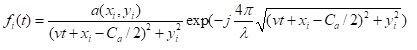(1)

where a(xi,yithe complex amplitude of dispersion i-oh a point, Ca length of the synthesized aperture, t-the current time, v-the constant speed of movement of the receiver with a radiator of rather Tomography object,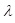-the wavelength of the probing signal.

Complex amplitude of the signal reflected from all object consisting of the I points it is possible to present in the form the sums of reflections from all points of object.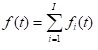(2)

where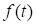the complex amplitude of the total signal reflected from the all I points object.

To reconstruct the image of object on one registered trajectory Doppler signal, it is necessary to create basic trajectory signals from basic dot objects in advance.

And reference points need to be "placed" on different removal from a rectilinear trajectory on a perpendicular to it with some step, blocking all range of ranges in which the Tomography object settles down.

The complex amplitude of a basic signal corresponding to reflection from basic dot object with coordinates (0,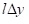), it is possible to write down as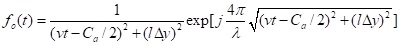(3)

where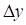- a step on a miss where the miss is understood as the shortest distance on a normal from a trajectory to a Tomography point,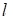- number of a step on a miss.

Then the set of one-dimensional mutual correlation functions between basic signals of f0 (t) and an object signal of f(t) can be presented in the form of function of two variables.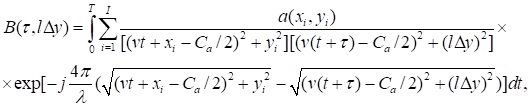(4)

where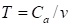- time of synthesizing of an aperture.

The ratio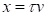allows to pass from temporary shift to spatial shift and to construct on expressions (4) two-dimensional function of dispersion of object in coordinates of a miss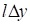and range on a trajectory.

It is necessary to pay attention that expression (4) is not two-dimensional mutual correlation function. It is a set of one-dimensional correlation functions for different ranges of a reference point.

However, it is presented by two-dimensional function (4). This function can be displayed on the plane and to construct a surface which will be similar to two-dimensional correlation function. If reset this surface the planes of different height and to combine the received sections, having painted them in different tone, then we will receive the tomogram of object.

Results of model reconstruction of the image of dot object are presented in Figure 5. The same results are yielded by reconstruction of section of a moving delay, Figure 2-a because the delay truncated down by the directional pattern of a piezo element (Figure 2-b), it is equivalent to dot object.

It is accepted to call a surface from correlation functions of Figure 5-b in literature transfer function of a point . It characterizes possibilities of the tomograph, in particular resolution of this method of reconstruction.

If reset a correlation surfaces the horizontal plane at the level of 0,7 from the maximum value, then we receive a circle diameter λ/5 which characterizes resolution of a method. Such resolution is known in literature as Rayleigh's limit .

Ideally for a tomography it would be desirable to have transfer function of a point in the form of Dirac's impulse. Such chance is given by multi-angles synthesis of an aperture. Let's explain essence of multi-angles synthesis of an aperture on the example of two-courses synthesis of an aperture. Let's register a trajectory Doppler signal, having provided the movement of a locator on a rectilinear trajectory.

After correlation processing of the registered trajectory signal basic trajectory signals from dot objects we will receive a correlation surface, that is transfer function of a point.

Then we will force a locator to move on other trajectory, a perpendicular initial trajectory. After correlation processing of again received trajectory signal we will have other correlation surface turned on 900 in relation to an initial surface.

Let's impose correlation matrixes of the turned and not turned surface and we will multiply the coincided elements of matrixes. Let's receive the resulting correlation surface which will correspond to two-courses aperture synthesis. It is presented in Figure 6. Thus, Figure 6 shows the result of superimposing two correlation surfaces, one of which is rotated by 90 relative to the original correlation surface.

When the values of the correlation functions were superimposed, they multiplied. As a result, two aperture synthesis of the aperture along two mutually perpendicular trajectories was obtained.

Multi-angles synthesis of an aperture is supposed to be used for elimination of an interference. To show how it is possible to eliminate an interference it is possible on the example of recovery of the image of multipoint object in the form of an obtuse angle of Figure 7 on one trajectory signal of Figure 9(the trajectory signal is shown on a Figure 8), on two signals from two orthogonal trajectories for Figure 10, an and four trajectory signals of Figure 10-b. At recovery of the image of object on four trajectory signals we will turn coordinate basis from orthogonal trajectories for the second couple from four trajectory signals on 45°. Figure 11.

Figure 9 shows the dense interferential picture hiding sixteen dot object. In Figure 10, the result of restoration of sixteen dot objects is presented in the form of an obtuse angle for orthogonal trajectories. Points of this object will defend from each other at distance 0,651

In Figure 10, b the image of sixteen dot objects in the form of an obtuse angle restored on four trajectory signals is presented. At the same time the basis of the second couple of orthogonal trajectories is turned concerning basis of the first couple of orthogonal trajectories on 45º (see Figure 11).

That at matrixes the bigger number of elements coincided, it is necessary to reduce distance between elements of one of matrixes in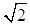time. For this purpose at registration and processing the step on a miss and a step along a trajectory also should be reduced intime. On a site of full overlapping of matrixes coincidence of elements reaches 50%. At the same time, it is necessary to multiply only the coincided elements of matrixes and to reject not coincident. The object on the turned matrix will hold the same position, as at not turned matrix.

Comparing the results presented in Figure 9 and Figure 10 it is possible to see that multi-angles synthesis of an aperture allows to weaken an interference.

5.2.      Synthesis of an Aperture on a Circular Trajectory

From this geometric diagram it is possible to determine the current distance R (t) from the moving locator to the i-th point of the object. It is supposed that the locator with the isotropic chart moves on a circular trajectory, irradiating the reflecting point with a monochromatic signal, and registers a trajectory Doppler signal of Figure 12 [5,6,7].

The distance from a Tomography point to a locator changes under the law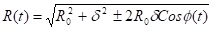(5)

Where,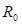- the radius of a circular trajectory on which the locator moves and is carried out aperture synthesis.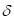- radial removal of a Tomography point from the center of synthesizing of an aperture.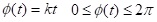- the current corner between radiuses of R0 and  at circular synthesizing of an aperture.

The trajectory signal of s(t) pays off on a formula:

for a case and without radial attenuation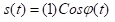(6)

for a case taking into account radial attenuation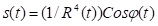(7)

where ,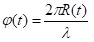,λ-wavelength

The Tomography object is representable multipoint model. In case of a large number of points the signal of s(t) reflected from them according to the principle of superposition can be calculated on a formula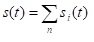(8)

Where n - total number of Tomography points of object,  i - serial number of a Tomography point of object, si(t) - the trajectory Doppler signal reflected from i-oh point of object and calculated on a formula (6) or (7).

For spatial selection of points of object, it is necessary to scan area of reconstruction of Figure 13 the reconstructed focal spot of the synthesized aperture for what the accepted Doppler signal of a look (8) is exposed to repeated correlation with the basic trajectory signals calculated for all reference points in a circular aperture of Figure 13.

In the case of Figure 13, the reference reflection points with known coordinates are uniformly distributed in the square reconstruction area, allowing to calculate the reference trajectory signals according to formulas (6) or (7).

If to take Fourier's transformation from a trajectory signal for a reference point, then the received range will define actually frequency characteristic of the reconstructed filter which is adjusted on k-yu a reference point.

That is, it is necessary to calculate mutually - the correlation Rk (t) functions of a look.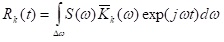(9)

where ,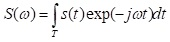(10)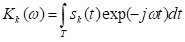(11)

In expressions (9), (10), (11) the following designations are accepted: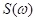- a range of the scanned signal (8)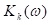- the frequency characteristic of the filter which is adjusted on k - yu a space point in a circle on which aperture synthesis is carried out,

sk(t) - the basic signal calculated on a formula (6) or (7) for one reference point located in k - oh areas of space. All square areas in the sum form a square, inscribed in a circle which defines area of reconstruction of Figure 13.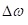- strip of frequencies of integration

T - time of synthesizing of an aperture, that is time of flight of object locator around.

Thus, process of creation of the image was reduced to repeated correlation (9) of a trajectory signal of a view (8) with the basic signals of a look (6) or (7) calculated at various ranges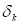, and development of the central counting mutually - correlation functions in the Cartesian coordinates recoded in tone symbols. For this purpose,  it is necessary to express as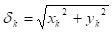where xk and yk the Cartesian coordinates of each k-oh point of a two-dimensional matrix of the image of Figure 13.

5.         Results of Reconstruction of Objects by Means of Circular Synthesis of the Aperture

Trajectory signals from dot objects at the movement of a locator are around presented in Figure 14. On a Figure 16 the top view on the correlation surfaces presented on a Figure 15 is shown.

6.         Problem of Circular Synthesis of the Aperture

The type of transfer function from above in essence is the tomogram of object. It is necessary to pay attention to a pedestal of transfer function (Figure 15). The pedestal is the basis of the transfer function, that is, the lower part of the correlation surface. It looks like a cone. The less pedestal, the result of a Tomography is better. At the level of a pedestal the interference is observed. Let's consider a pedestal in more detail than Figure 17.

Figure 17 consists of rings whose height grows as the diameter of the ring decreases. A cone is formed from the rings, passing into the delta function.

We see rings which it is possible to call "Airey's Rings". The reason of their emergence easily speaks. Circular synthesis of an aperture should be finished, having made one turn. We are forced to stop a moving locator and to tear off a trajectory signal rice 14. Break of a trajectory signal is followed by manifestation of effect of Gibbs, that is not the steady state is followed by refluctuations. These refluctuations eventually are made out in Airey's rings.

This problem is designated in D. Mensah  Predlozhennoye's work by it the solution disposal of rings consists in applying multi frequency sounding, that is to leave from a coherent tomography.

Until now circular synthesis of an aperture without radial attenuation of a trajectory signal of Figure 18, a was considered. In Figure 18-b the same point, under the same conditions, but taking into account radial attenuation of a trajectory signal according to a formula (7).

At the accounting of radial attenuation, the so-called system of automatic adjustment of strengthening which after correlation processing increased amplitude of responses according to remoteness of a point from a trajectory of synthesizing of an aperture was provided in the program of calculation.

Comparing Figure 18-a and Figure 18-b can see how at the accounting of radial attenuation, transfer function of a point in section the horizontal plane takes a form of the eight which is characteristic of linear aperture synthesis [2,3]. This result is obvious as for the point remote from the center and brought closer to a trajectory, at the accounting of radial attenuation, effective synthesizing of an aperture happens only on a piece of an arch of a circle, approximate to a point which can be interpreted as a piece of a rectilinear trajectory. That is transition to rectilinear synthesis of an aperture is observed.

7.         Ring Ranges of Transfer Functions

Interesting property of transfer functions is noticed. Let's take two-dimensional transformation of Fourier from transfer function of Figure 15. As a result of such transformation ring ranges turn out . The ring spectrum is shown in Figure 19-a. Figure 19-b shows a top view of the circular spectrum depicted in Figure 19-a. Figure 19-c shows the phase portrait of the ring spectrum.

They are a little studied and very few people apply them to processing of transfer functions. Ring ranges are received in 80-x years . Dean Mensah registered ring ranges, and received transfer function by the return two-dimensional transformation of Fourier from a ring range.

8.       Conclusion

Aperture synthesis at small range opens great opportunities, in particular in a tomography. The monochromatic signal allows to avoid dispersive distortions at reconstruction of the image of objects. Resolution of a method allows to reach Rayleigh's limit, that is λ/5. Changing wavelength, it is possible to operate resolution of the tomograph.

To use these remarkable qualities of aperture synthesis it is necessary to overcome many problems. Problems arise constantly in process of advance in realization of a laboratory sample. For example, it is possible to face a problem of reflection of the ultrasonic probing signal from the superficial wave left by the sonar antennas moving in water. The solution is considered in .

The arising problems are overcome in process of their identification. Help the solution of problems of achievement in technologies, and development of the computer equipment.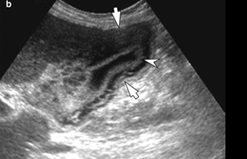Figure 1: Ultrasonography image of a gall bladder.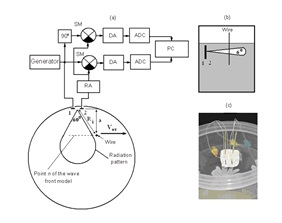Figures 2(a-c): a) the Geometrical and function chart of data collection (the top view in the equatorial plane), b) The directional pattern of piezo elements 1 and 2 in the meridional plane, c) a design of piezo elements 1 and 2. If it is required to realize circular synthesis of an aperture, then fix Tomography object, for example a delay in motionless situation, and begin to rotate the tank with water with a constant speed. At the same time piezo elements, (that is ultrasonic antennas) the tank walls attached to internal with water will make circular movement around Tomography object, being in the water environment.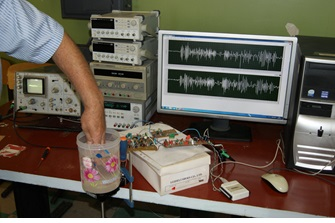Figure 3: Ultrasonic experimental installation for Tomography of objects in water. The white square from the material absorbing ultrasound on a tank sidewall from the inside contains two piezoceramic elements which carry out function of ultrasonic antennas of a Doppler locator.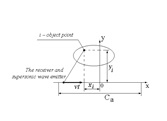Figure 4: Geometry, the Tomography explaining process.